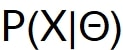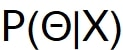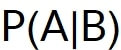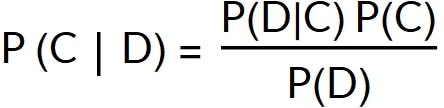## What is a Bayesian estimator?

An estimator is Bayesian if it uses the Bayes theorem to predict the most likely class of some observed data.

Because the class of data is an unknown parameter and not a random variable, it is not possible to express the probability of that class using the standard concept of probability.

Bayesian probability uses a different notion of probability which quantifies our state of knowledge about the truth of an assertion.

With standard probability, the conditional probability of some random variable X, assuming some value of an unknown parameter theta, cannot be inverted.

You can write the following formula because X is a random variable:But you cannot write the following formula, because theta is not a random variable, but is an unknown parameter:Bayesian probability allows you to express the probability that some logical assertions are true.

This means that there is a symmetry in the conditional probability of A assuming B which is expressed with following formula:A and B are both logical assertions. Therefore, the previous formula can be inverted using the Bayes theorem to get the probability of B assuming A.

If A and B are the observed data D and the class of the data C, the Bayes theorem allows you to relate what you know about the data and the classes.

Bayes theorem allows you to compute the most likely class for some observed data, if you know something about how the data depends on the classes.

The probability of a class assuming some observed data is expressed as P(C|D). The probability of the data assuming some class C is expressed as P(D|C).

The Bayes theorem is shown here:For the naive gaussian Bayesian classifier, the data, D, is a vector of samples. We assume that the samples are independent and that they follow a Gaussian distribution.

The parameters of the gaussian for each class will be computed during the training of the Python classifier. For each gaussian, the mean and standard deviation is computed.

Also, if some information about the class is available, and some classes are more or less likely, then this knowledge is encoded in the prior probability P(C).

The Python code also returns a value epsilon, which is needed for numerical accuracy.